Courses
Courses for Kids
Free study material
Offline Centres
More

# NCERT Solutions for Class 9 Maths Chapter 7 - ExerciseLast updated date: 30th Nov 2023
Total views: 532.8k
Views today: 14.32k

## NCERT Solutions for Class 9 Maths Chapter 7 Triangles (Ex 7.3) Exercise 7.3

Students will study in detail triangles and their various properties in NCERT solutions for class 9 maths chapter 7 exercise 7.3. The chapter introduces the definition of a triangle, congruence of triangles, inequalities in a triangle, rules of congruence, and more. The chapter explains some rules or criteria for the congruence of triangles. It covers Side- Angle- Side Congruence Rule, Angle- Side- Side Congruence Rule, and Angle- Angle- Side Congruence Rule. Apart from it, other properties of an isosceles triangle, theorems related to triangles, and more concepts are explained in detail in ch 7 Maths NCERT Solutions Class 9 ex 7.3. Students can also avail of NCERT Solutions for Class 9 Science from our website.

Watch videos on
NCERT Solutions for Class 9 Maths Chapter 7 - ExerciseTRIANGLES L-1 (𝐂𝐨𝐧𝐠𝐫𝐮𝐞𝐧𝐜𝐞 𝐨𝐟 𝐓𝐫𝐢𝐚𝐧𝐠𝐥𝐞𝐬 & 𝐂𝐫𝐢𝐭𝐞𝐫𝐢𝐚 𝐟𝐨𝐫 𝐂𝐨𝐧𝐠𝐫𝐮𝐞𝐧𝐜𝐞 𝐨𝐟 𝐓𝐫𝐢𝐚𝐧𝐠𝐥𝐞𝐬) CBSE 9 Maths -Term 1
Vedantu 9&10
SubscribeShare
3.1K likes
89.7K Views
2 years agoTriangles in 30 Minutes | Crash Course | Maths | Gopal Sir | Vedantu Class 9
Vedantu 9&10
10.7K likes
315.3K Views
4 years ago
Play Quiz

## Access NCERT Solutions for Math Class 9 Chapter 7 – Triangles

### Exercise (7.1)

1. In quadrilateral $\mathbf{\text{ACBD,AC= AD}}$ and $\mathbf{\text{AB}}$ bisects $\mathbf{\angle \text{A}}$ (See the given figure). Show that $\mathbf{\text{ }\!\!\Delta\!\!\text{ ABC}\cong \text{ }\!\!\Delta\!\!\text{ ABD}}$. What can you say about $\mathbf{\text{BC}}$ and $\mathbf{\text{BD}}$?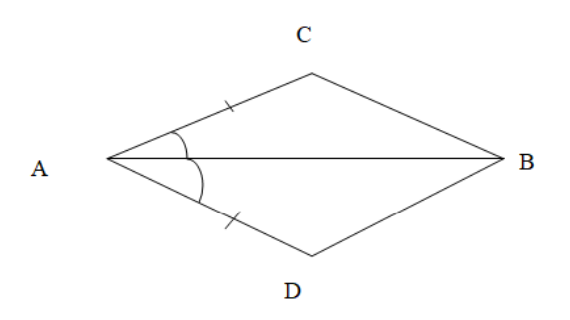Ans: In $\text{ }\!\!\Delta\!\!\text{ ABC}$ and $\text{ }\!\!\Delta\!\!\text{ ABD}$,

$\text{AC=AD}$                                   (Given)

$\angle \text{CAB=}\angle \text{DAB}$                        ($\text{AB}$ bisects $\angle \text{A}$)

$\text{AB=AB}$                                   (Common)

Therefore, $\text{ }\!\!\Delta\!\!\text{ ABC}\cong \text{ }\!\!\Delta\!\!\text{ ABD}$  (By $\text{SAS}$ congruence rule)

Therefore, $\text{BC= BD}$                 (By $\text{CPCT}$)

Therefore, $\text{BC}$ and $\text{BD}$are of equal lengths.

2. In quadrilateral $\mathbf{\text{ABCD}}$, $\mathbf{\text{AD = BC}}$ and $\mathbf{\angle \text{DAB =}\angle \text{CBA}}$ (See the given figure). Prove that: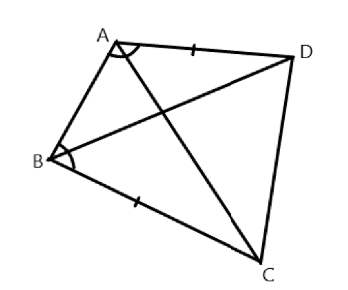(i) $\mathbf{\text{ }\!\!\Delta\!\!\text{ ABD}\cong \text{ }\!\!\Delta\!\!\text{ BAC}}$

Ans: In $\text{ }\!\!\Delta\!\!\text{ ABD}$ and $\text{ }\!\!\Delta\!\!\text{ BAC}$,

$\text{AD=BC}$                  (Given)

$\angle \text{DAB=}\angle \text{CBA}$      (Given)

$\text{AB= BA}$                 (Common)

Therefore, $\text{ }\!\!\Delta\!\!\text{ ABD}\cong \text{ }\!\!\Delta\!\!\text{ BAC}$

(ii) $\mathbf{\text{ }\!\!~\!\!\text{ BD = AC}}$

Ans: Therefore, $\text{BD = AC}$                 (By $\text{CPCT}$)

(iii) $\mathbf{\angle \text{ABD=}\angle \text{BAC}}$

Ans: Therefore, $\angle \text{ABD=}\angle \text{BAC}$     (By $\text{CPCT}$)

3. $\mathbf{\text{AD}}$ and $\mathbf{\text{BC}}$ are equal perpendiculars to a line segment $\mathbf{\text{AB}}$ (See the given figure). Show that $\mathbf{\text{CD}}$ bisects $\mathbf{\text{AB}}$.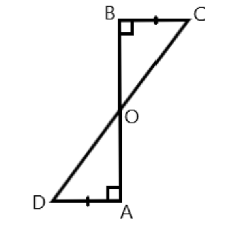Ans: In $\text{ }\!\!\Delta\!\!\text{ BOC}$ and $\text{ }\!\!\Delta\!\!\text{ AOD}$,

$\angle \text{BOC=}\angle \text{AOD}$                              (Vertically opposite angles)

$\angle \text{CBO=}\angle \text{DAO}$                              (Each $\text{90 }\!\!{}^\text{o}\!\!\text{ }$)

$\text{BC= AD }$                                        (Given)

Therefore, $\text{ }\!\!\Delta\!\!\text{ BOC}\cong \text{ }\!\!\Delta\!\!\text{ AOD}$            (By $\text{AAS}$ congruence rule)

Therefore, $\text{BO =AO}$                       (By $\text{CPCT}$)

Hence, $\text{CD}$ bisects $\text{AB}$.

4. Lines $\mathbf{\text{l}}$ and $\mathbf{\text{m}}$ are two parallel lines intersected by another pair of parallel lines $\mathbf{\text{p}}$ and $\mathbf{\text{q}}$ (see the given figure). Show that $\mathbf{\text{ }\!\!\Delta\!\!\text{ ABC}\cong \text{ }\!\!\Delta\!\!\text{ CDA}}$.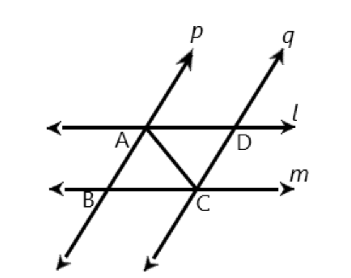Ans: In $\text{ }\!\!\Delta\!\!\text{ ABC}$ and $\text{ }\!\!\Delta\!\!\text{ CDA}$,

$\angle \text{BAC=}\angle \text{DCA}$                        (Alternate interior angles)

$\text{AC=CA}$                                   (Common)

$\angle \text{BCA=}\angle \text{DAC}$                        (Alternate interior angles)

Therefore, $\text{ }\!\!\Delta\!\!\text{ ABC}\cong \text{ }\!\!\Delta\!\!\text{ CDA}$     (By $\text{ASA}$ congruence rule)

5. Line $\mathbf{l}$ is the bisector of an angle $\mathbf{\angle \text{A}}$ and $\mathbf{B}$ is any point on $\mathbf{l}$. $\mathbf{BP}$ and $\mathbf{BQ}$ are perpendicular from $\mathbf{B}$ to the arms of $\mathbf{\angle \text{A}}$ (see the figure). Show that: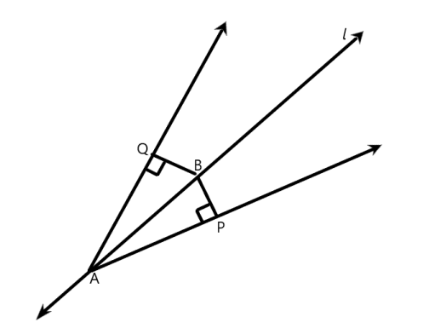(i) $\mathbf{\text{ }\!\!\Delta\!\!\text{ APB}\cong \text{ }\!\!\Delta\!\!\text{ AQB}}$

Ans: In $\text{ }\!\!\Delta\!\!\text{ APB}$ and $\text{ }\!\!\Delta\!\!\text{ AQB}$,

$\angle \text{APB=}\angle \text{AQB}$                     (Each $\text{90 }\!\!{}^\text{o}\!\!\text{ }$)

$\angle \text{PAB=}\angle \text{QAB}$                     ($\text{l}$ is the angle bisector of $\angle \text{A}$)

$\text{AB=AB}$                                (Common)

Therefore, $\text{ }\!\!\Delta\!\!\text{ APB}\cong \text{ }\!\!\Delta\!\!\text{ AQB}$  (By $\text{AAS}$ congruence rule)

(ii) $\mathbf{\text{BP=BQ}}$ or $\mathbf{B}$ is equidistant from the arms of $\mathbf{\angle \text{A}}$.

Ans: $\text{BP=BQ}$ (By $\text{CPCT}$)

Or we can say that $\text{B}$ is equidistant from the arms of $\angle \text{A}$.

6. In the given figure, $\mathbf{\text{AC=AE,AB=AD}}$ and $\mathbf{\angle \text{BAD=}\angle \text{EAC}}$. Show that $\mathbf{\text{BC=DE}}$.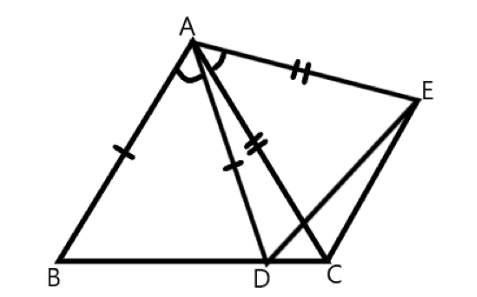Ans: It is given that

$\angle \text{BAD=}\angle \text{EAC}$

$\angle \text{BAD+}\angle \text{DAC=}\angle \text{EAC+}\angle \text{DAC}$

$\angle \text{BAC=}\angle \text{DAE}$

In $\text{ }\!\!\Delta\!\!\text{ BAC}$ and $\text{ }\!\!\Delta\!\!\text{ DAE}$,

$\text{AB=AD}$                                   (Given)

$\angle \text{BAC=}\angle \text{DAE}$                        (Proved above)

$\text{AC=AE}$                                   (Given)

Therefore, $\text{ }\!\!\Delta\!\!\text{ BAC}\cong \text{ }\!\!\Delta\!\!\text{ DAE}$     (By $\text{SAS}$ congruence rule)

Therefore, $\text{BC = DE}$                (By $\text{CPCT}$)

7. $\mathbf{AB}$ is a line segment and $\mathbf{P}$ is its mid-point. $\mathbf{D}$ and $\mathbf{E}$ are points on the same side of $AB$ such that

$\mathbf{\angle \text{BAD=}\angle \text{ABE}}$ and $\mathbf{\angle \text{EPA=}\angle \text{DPB}}$ (See the given figure). Show that: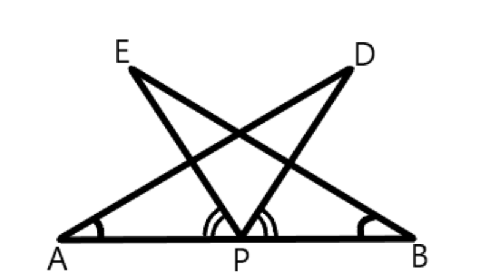(i) $\mathbf{\text{ }\!\!\Delta\!\!\text{ DAP}\cong \text{ }\!\!\Delta\!\!\text{ EBP}}$

Ans: It is given that

$\angle \text{EPA=}\angle \text{DPB}$

$\angle \text{EPA+}\angle \text{DPE =}\angle \text{DPB +}\angle \text{DPE}$

Therefore, $\angle \text{DPA=}\angle \text{EPB}$.

In $\text{ }\!\!\Delta\!\!\text{ DAP}$ and $\text{ }\!\!\Delta\!\!\text{ EBP}$,

$\angle \text{DAP=}\angle \text{EBP}$                          (Given)

$\text{AP=BP}$                                     ($\text{P}$ is midpoint of $\text{AB}$)

$\angle \text{DPA=}\angle \text{EPB}$                          (From above)

Therefore, $\text{ }\!\!\Delta\!\!\text{ DAP}\cong \text{ }\!\!\Delta\!\!\text{ EBP}$       (By $\text{ASA}$ congruence rule)

(ii) $\mathbf{\text{AD=BE}}$

Ans: $AD\text{ }=BE$      (By $\text{CPCT}$)

8. In right triangle $\mathbf{\text{ABC}}$, right angled at $\mathbf{\text{C}}$, $\mathbf{\text{M}}$ is the mid-point of hypotenuse $\mathbf{\text{AB}}$. $\mathbf{\text{C}}$ is joined to $\mathbf{\text{M}}$ and produced to a point $\mathbf{\text{D}}$ such that $\mathbf{\text{DM=CM}}$. Point $\mathbf{\text{D}}$ is joined to point $\mathbf{\text{B}}$ (see the given figure). Show that: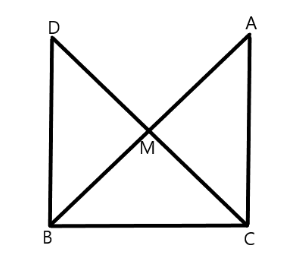(i) $\mathbf{\text{ }\!\!\Delta\!\!\text{ AMC}\cong \text{ }\!\!\Delta\!\!\text{ BMD}}$

Ans: In $\text{ }\!\!\Delta\!\!\text{ AMC}$ and $\text{ }\!\!\Delta\!\!\text{ BMD}$,

$\text{AM=BM}$                                   ($\text{M}$ is the midpoint of $\text{AB}$)

$\angle \text{AMC=}\angle \text{BMD}$                        (Vertically opposite angles)

$\text{CM=DM}$                                   (Given)

Therefore, $\text{ }\!\!\Delta\!\!\text{ AMC}\cong \text{ }\!\!\Delta\!\!\text{ BMD}$     (By $\text{SAS}$ congruence rule)

(ii) $\mathbf{\angle \text{DBC}}$ is a right angle

Ans: $\angle \text{ACM=}\angle \text{BDM}$

Since $\angle \text{ACM}$ and $\angle \text{BDM}$ are alternate interior angles, line $\text{DB }\!\!|\!\!\text{ }\!\!|\!\!\text{ AC}$.

So,

$\angle \text{DBC+}\angle \text{ACB=180 }\!\!{}^\text{o}\!\!\text{ }$

$\angle \text{DBC+ 90 }\!\!{}^\text{o}\!\!\text{ = 180 }\!\!{}^\text{o}\!\!\text{ }$

Therefore, $\angle \text{DBC= 90 }\!\!{}^\text{o}\!\!\text{ }$

(iii) $\mathbf{\text{ }\!\!\Delta\!\!\text{ DBC}\cong \text{ }\!\!\Delta\!\!\text{ ACB}}$

Ans: In $\text{ }\!\!\Delta\!\!\text{ DBC}$ and $\text{ }\!\!\Delta\!\!\text{ ACB}$,

$\text{DB=AC}$                                      (Already proved)

$\angle \text{DBC=}\angle \text{ACB}$                          (Each $\text{90 }\!\!{}^\text{o}\!\!\text{ }$)

$\text{BC=CB}$                                      (Common)

Therefore, $\text{ }\!\!\Delta\!\!\text{ DBC}\cong \text{ }\!\!\Delta\!\!\text{ ACB}$        (By $\text{SAS}$ congruence rule)

(iv) $\mathbf{\text{CM=}\frac{\text{1}}{\text{2}}\text{AB}}$

Ans: Since, $\text{ }\!\!\Delta\!\!\text{ DBC}\cong \text{ }\!\!~\!\!\text{ }\!\!\Delta\!\!\text{ ACB}$.

$\text{AB=DC}$                                         (By $\text{CPCT}$)

$\text{AB=2CM}$

Therefore, $\text{CM=}\frac{\text{1}}{\text{2}}\text{AB}$.

### Exercise (7.2)

1. In an isosceles triangle $\mathbf{ABC}$, with $\mathbf{\text{AB=AC}}$, the bisectors of $\mathbf{\angle B}$ and $\mathbf{\angle C}$ intersect each other at $\mathbf{O}$. Join $\mathbf{A}$ to $\mathbf{O}$. Show that: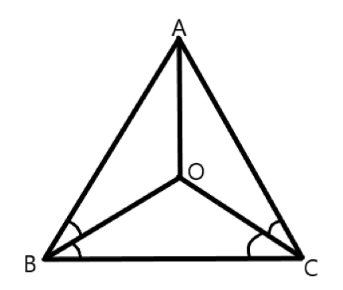(i) $\mathbf{\text{OB=OC}}$

Ans: It is given that in triangle $ABC$, $\text{AB=AC}$.

$\angle \text{ACB=}\angle \text{ABC}$                     (Angles opposite to equal sides of a triangle are equal)

$\frac{\text{1}}{\text{2}}\angle \text{ACB=}\frac{\text{1}}{\text{2}}\angle \text{ABC}$

$\angle \text{OCB= }\angle \text{OBC}$

Therefore, $\text{OB=OC}$.           (Sides opposite to equal angles of a triangle are equal)

(ii) $\mathbf{\text{AO}}$ bisects $\mathbf{\angle \text{A}}$

Ans: In $\text{ }\!\!\Delta\!\!\text{ OAB}$ and $\text{ }\!\!\Delta\!\!\text{ OAC}$,

$\text{AO=AO}$                                           (Common)

$\text{AB=AC}$                                            (Given)

$\text{OB=OC}$                                            (Proved above)

Therefore, $\text{ }\!\!\Delta\!\!\text{ OAB}\cong \text{ }\!\!\Delta\!\!\text{ OAC}$              (By $\text{SSS}$ congruence rule)

And, $\angle \text{BAO=}\angle \text{CAO}$                        (By $\text{CPCT}$)

Therefore, $\text{AO}$ bisects $\angle \text{A}$.

2. In $\mathbf{\text{ }\!\!\Delta\!\!\text{ ABC}}$, $\mathbf{\text{AD}}$ is the perpendicular bisector of $\mathbf{\text{BC}}$ (see the given figure). Show that $\mathbf{\text{ }\!\!\Delta\!\!\text{ ABC}}$ is an isosceles triangle in which $\mathbf{\text{AB=AC}}$.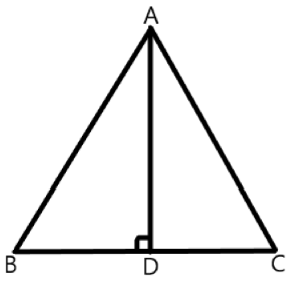Ans: In $\text{ }\!\!\Delta\!\!\text{ ADC}$ and $\text{ }\!\!\Delta\!\!\text{ ADB}$,

$\text{AD=AD}$                                         (Common)

$\angle \text{ADC=}\angle \text{ADB}$                              (Each $\text{90 }\!\!{}^\text{o}\!\!\text{ }$)

$\text{CD=BD}$                                          ($\text{AD}$ is perpendicular bisector of $\text{BC}$)

Therefore, $\text{ }\!\!\Delta\!\!\text{ ADC}\cong \text{ }\!\!\Delta\!\!\text{ ADB}$            (By $\text{SAS}$ congruence rule)

And, $\text{AB= AC}$

Hence, $\text{ }\!\!\Delta\!\!\text{ ABC}$ is an isosceles triangle in which $\text{AB=AC}$.

3. ABC is an isosceles triangle in which altitudes BE and CF are drawn to equal sides AC and AB respectively (see the given figure). Show that these altitudes are equal.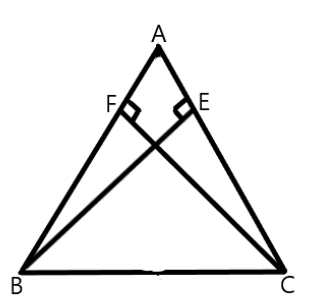Ans: In $\text{ }\!\!\Delta\!\!\text{ AEB}$ and $\text{ }\!\!\Delta\!\!\text{ AFC}$,

$\angle \text{AEB=}\angle \text{AFC}$                              (Each $\text{90 }\!\!{}^\text{o}\!\!\text{ }$)

$\angle \text{A= }\angle \text{A}$                                       (Common)

$\text{AB=AC}$                                         (Given)

Therefore, $\text{ }\!\!\Delta\!\!\text{ AEB}\cong \text{ }\!\!\Delta\!\!\text{ AFC}$            (By $\text{AAS}$ congruence rule)

Therefore, $\text{BE=CF}$                        (By $\text{CPCT}$)

4. ABC is a triangle in which altitudes BE and CF to sides AC and AB are equal (see the given figure). Show that(i) $\mathbf{\text{ }\!\!\Delta\!\!\text{ ABE }\cong \text{ }\!\!\Delta\!\!\text{ ACF}}$

Ans: In $\text{ }\!\!\Delta\!\!\text{ ABE}$ and $\text{ }\!\!\Delta\!\!\text{ ACF}$,

$\angle \text{ABE=}\angle \text{ACF}$                            (Each $\text{90 }\!\!{}^\text{o}\!\!\text{ }$)

$\angle \text{A= }\angle \text{A}$                                      (Common)

$\text{BE= CF}$                                        (Given)

Therefore, $\text{ }\!\!\Delta\!\!\text{ ABE}\cong \text{ }\!\!\Delta\!\!\text{ ACF}$   (By $\text{AAS}$ congruence rule)

(ii) $\mathbf{\text{AB=AC}}$, i.e., $\mathbf{\text{ABC}}$ is an isosceles triangle.

Ans: Since, $\text{ }\!\!\Delta\!\!\text{ ABE}\cong \text{ }\!\!\Delta\!\!\text{ ACF}$.

Therefore, $AB=AC$                    (By $\text{CPCT}$)

5. $\mathbf{\text{ABC}}$ and $\mathbf{\text{DBC}}$ are two isosceles triangles on the same base $\mathbf{\text{BC}}$ (see the given figure). Show that $\mathbf{\angle \text{ABD=}\angle \text{ACD}}$.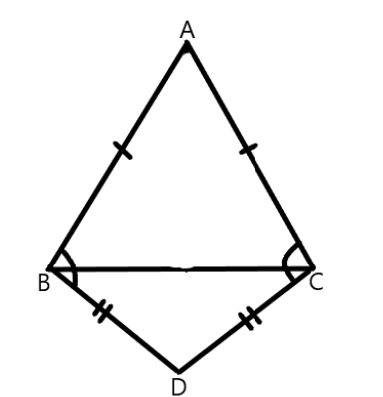Ans: Let us join $AD$.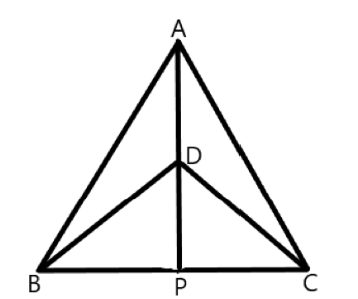In $\text{ }\!\!\Delta\!\!\text{ ABD}$ and $\text{ }\!\!\Delta\!\!\text{ ACD}$,

$\text{AB=AC}$                                          (Given)

$\text{BD=CD}$                                          (Given)

$\text{AD=AD}$                                         (Common side)

Therefore, $\text{ }\!\!\Delta\!\!\text{ ABD}\cong \text{ }\!\!\Delta\!\!\text{ ACD}$           (By $\text{SSS}$ congruence rule)

Therefore, $\angle \text{ABD= }\angle \text{ACD}$           (By $CPCT$)

6. $\mathbf{\text{ }\!\!\Delta\!\!\text{ ABC}}$ is an isosceles triangle in which $\mathbf{\text{AB=AC}}$. Side $\mathbf{BA}$ is produced to $\mathbf{D}$ such that $\mathbf{\text{AD=AB}}$ (see the given figure). Show that $\mathbf{\angle \text{BCD}}$ is a right angle.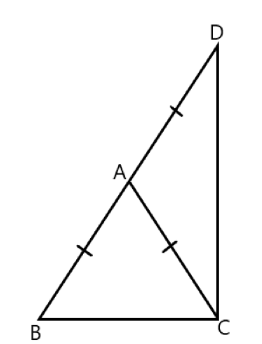Ans: In $\text{ }\!\!\Delta\!\!\text{ ABC}$,

$\text{AB=AC}$                                                             (Given)

Therefore, $\angle \text{ACB=}\angle \text{ABC}$                                (Angles opposite to equal sides of a triangle are equal)

In $\text{ }\!\!\Delta\!\!\text{ ACD}$,

$\text{AC=AD}$

Therefore, $\angle \text{ADC=}\angle \text{ACD}$                               (Angles opposite to equal sides of a triangle are equal)

In $\text{ }\!\!\Delta\!\!\text{ BCD}$,

$\angle \text{ABC+ }\angle \text{BCD+ }\angle \text{ADC=180 }\!\!{}^\text{o}\!\!\text{ }$                        (Angle sum property of a triangle)

$\angle \text{ACB+}\angle \text{ACB+}\angle \text{ACD+}\angle \text{ACD=180 }\!\!{}^\text{o}\!\!\text{ }$

$\text{2}\left( \angle \text{ACB+}\angle \text{ACD} \right)\text{=180 }\!\!{}^\text{o}\!\!\text{ }$

$\text{2}\left( \angle \text{BCD} \right)\text{= 180 }\!\!{}^\text{o}\!\!\text{ }$

Therefore, $\angle \text{BCD = 90 }\!\!{}^\text{o}\!\!\text{ }$.

7. $\mathbf{\text{ }\!\!\Delta\!\!\text{ ABC}}$ is a right angled triangle in which $\mathbf{\angle \text{A=90 }\!\!{}^\text{o}\!\!\text{ }}$ and $\mathbf{\text{AB=AC}}$. Find $\mathbf{\angle \text{B}}$ and $\mathbf{\angle \text{C}}$.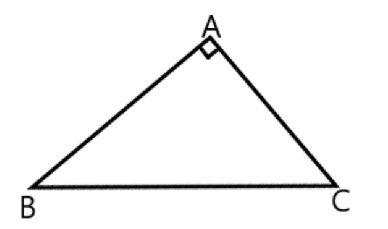Ans: It is given that:

$\text{AB=AC}$

Therefore, $\angle \text{C= }\angle \text{B}$

In $\text{ }\!\!\Delta\!\!\text{ ABC}$,

$\angle \text{A+}\angle \text{B+}\angle \text{C= 180 }\!\!{}^\text{o}\!\!\text{ }$

$\text{90 }\!\!{}^\text{o}\!\!\text{ +}\angle \text{B+}\angle \text{C =180 }\!\!{}^\text{o}\!\!\text{ }$

$\text{90 }\!\!{}^\text{o}\!\!\text{ +}\angle \text{B+ }\angle \text{B= 180 }\!\!{}^\text{o}\!\!\text{ }$

$\text{2}\angle \text{B =90 }\!\!{}^\text{o}\!\!\text{ }$

$\angle \text{B =45 }\!\!{}^\text{o}\!\!\text{ }$

Therefore, $\angle \text{B =}\angle \text{C=45 }\!\!{}^\text{o}\!\!\text{ }$

8. Show that the angles of an equilateral triangle are $\mathbf{\text{6}{{\text{0}}^{\text{o}}}}$ each.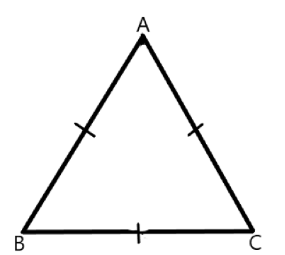Ans: Let us consider that $\text{ }\!\!\Delta\!\!\text{ ABC}$ is an equilateral triangle.

So,

$\text{AB=BC=AC}$

Therefore,

$\angle \text{C= }\angle \text{B}$

$\angle \text{B= }\angle \text{A}$

Hence, $\angle \text{A=}\angle \text{B =}\angle \text{C}$

In $\text{ }\!\!\Delta\!\!\text{ ABC}$,

$\angle \text{A+}\angle \text{B +}\angle \text{C =180 }\!\!{}^\circ\!\!\text{ }$

$\text{3}\angle \text{A=180 }\!\!{}^\circ\!\!\text{ }$

$\text{3}\angle \text{A=180 }\!\!{}^\circ\!\!\text{ }$

Therefore, $\angle \text{A=}\angle \text{B =}\angle \text{C=6}0{}^\circ$

Hence, in an equilateral triangle, all interior angles are of measure $\text{6}{{\text{0}}^{\text{o}}}$.

### Exercise (7.3)

1. $\mathbf{\text{ }\!\!\Delta\!\!\text{ ABC}}$ and $\mathbf{\text{ }\!\!\Delta\!\!\text{ DBC}}$ are two isosceles triangles on the same base $\mathbf{\text{BC}}$ and vertices $\mathbf{\text{A}}$ and $\mathbf{\text{D}}$ are on the same side of $\mathbf{\text{BC}}$ (see the given figure). If $\mathbf{\text{AD}}$ is extended to intersect $\mathbf{\text{BC}}$ at $\mathbf{\text{P}}$, show that:(i) $\mathbf{\text{ }\!\!\Delta\!\!\text{ ABD}\cong \text{ }\!\!\Delta\!\!\text{ ACD}}$

Ans: In $\text{ }\!\!\Delta\!\!\text{ ABD}$ and $\text{ }\!\!\Delta\!\!\text{ ACD}$,

$\text{AB=AC}$                                           (Given)

$\text{BD=CD}$                                           (Given)

$\text{AD=AD}$                                          (Common)

Therefore, $\text{ }\!\!\Delta\!\!\text{ ABD}\cong \text{ }\!\!\Delta\!\!\text{ ACD}$         (By $\text{SSS}$ rule)

$\angle \text{BAD=}\angle \text{CAD}$                            (By $CPCT$)

$\angle \text{BAP=}\angle \text{CAP}$

(ii) $\mathbf{\text{ }\!\!\Delta\!\!\text{ ABP }\cong \text{ }\!\!\Delta\!\!\text{ ACP}}$

Ans: In $\text{ }\!\!\Delta\!\!\text{ ABP}$ and $\text{ }\!\!\Delta\!\!\text{ ACP}$,

$\text{AB=AC}$                                         (Given)

$\angle \text{BAP = }\angle \text{CAP}$

$\text{AP= AP}$                                         (Common)

Therefore, $\text{ }\!\!\Delta\!\!\text{ ABP }\cong \text{ }\!\!\Delta\!\!\text{ ACP}$          (By $\text{SAS}$ congruence rule)

Therefore, $\text{BP=CP}$                         (By $CPCT$)

(iii) $\mathbf{\text{AP}}$ bisects $\mathbf{\angle \text{A}}$ as well as $\mathbf{\angle \text{D}}$.

Ans: Since, $\angle \text{BAP=}\angle \text{CAP}$,

Therefore, $\text{AP}$ bisects $\angle \text{A}$.

In $\text{ }\!\!\Delta\!\!\text{ BDP}$ and $\text{ }\!\!\Delta\!\!\text{ CDP}$,

$\text{BD= CD}$                                      (Given)

$\text{DP=DP}$                                        (Common)

$\text{BP= CP}$

Therefore, $\text{ }\!\!\Delta\!\!\text{ BDP}\cong \text{ }\!\!\Delta\!\!\text{ CDP}$       (By $\text{SSS}$ congruence rule)

Therefore, $\angle \text{BDP=}\angle \text{CDP}$        (By $\text{CPCT}$)

Hence, $\text{AP}$ bisects $\angle \text{D}$.

(iv) $\mathbf{\text{AP}}$ is the perpendicular bisector of $\mathbf{\text{BC}}$.

Ans: Since $\text{ }\!\!\Delta\!\!\text{ BDP}\cong \text{ }\!\!\Delta\!\!\text{ CDP}$,

Therefore,

$\angle \text{BPD =}\angle \text{CPD}$                           (By $\text{CPCT}$)

$\angle \text{BPD+}\angle \text{CPD=18}{{\text{0}}^{\text{o}}}$                   (Linear pair of angles)

$\angle \text{BPD +}\angle \text{BPD = 18}{{\text{0}}^{\text{o}}}$                (Since $\angle \text{BDP=}\angle \text{CDP}$)

$\text{2}\angle \text{BPD=18}{{\text{0}}^{\text{o}}}$

$\angle \text{BPD = 9}{{\text{0}}^{\text{o}}}$

Hence $\text{AP}$ is the perpendicular bisector of $\text{BC}$.

2. $\mathbf{AD}$ is an altitude of an isosceles triangles $\mathbf{ABC}$ in which $\mathbf{\text{AB=AC}}$. Show that: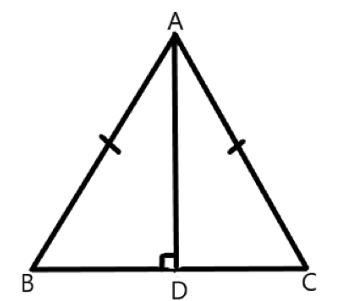(i) $\mathbf{AD}$ bisects $\mathbf{\text{BC}}$

Ans: In $\text{ }\!\!\Delta\!\!\text{ BAD}$ and $\text{ }\!\!\Delta\!\!\text{ CAD}$,

$\angle \text{ADB= }\angle \text{ADC}$                           (Each $\text{90 }\!\!{}^\text{o}\!\!\text{ }$)

$\text{AB=AC}$                                        (Given)

$\text{AD=AD}$                                       (Common)

Therefore, $\text{ }\!\!\Delta\!\!\text{ BAD }\cong \text{ }\!\!\Delta\!\!\text{ CAD}$        (By $\text{RHS}$ congruence rule)

Therefore, $\text{BD = CD}$                   (By $\text{CPCT}$)

Hence, $\text{AD}$ bisects $\text{BC}$.

(ii) $\mathbf{AD}$ bisects $\mathbf{\angle \text{A}}$.

Ans: Also, $\angle \text{BAD=}\angle \text{CAD}$           (By $\text{CPCT}$)

Hence, $AD$ bisects $BC$.

Therefore, $AD$ bisects $\angle A$.

3. Two sides $\mathbf{\text{AB}}$ and $\mathbf{\text{BC}}$ and median $\mathbf{\text{AM}}$ of one triangle $\mathbf{\text{ABC}}$ are respectively equal to sides $\mathbf{\text{PQ}}$ and $\mathbf{\text{QR}}$ and median $\mathbf{\text{PN}}$ of $\mathbf{\text{ }\!\!\Delta\!\!\text{ PQR}}$ (see the given figure). Show that: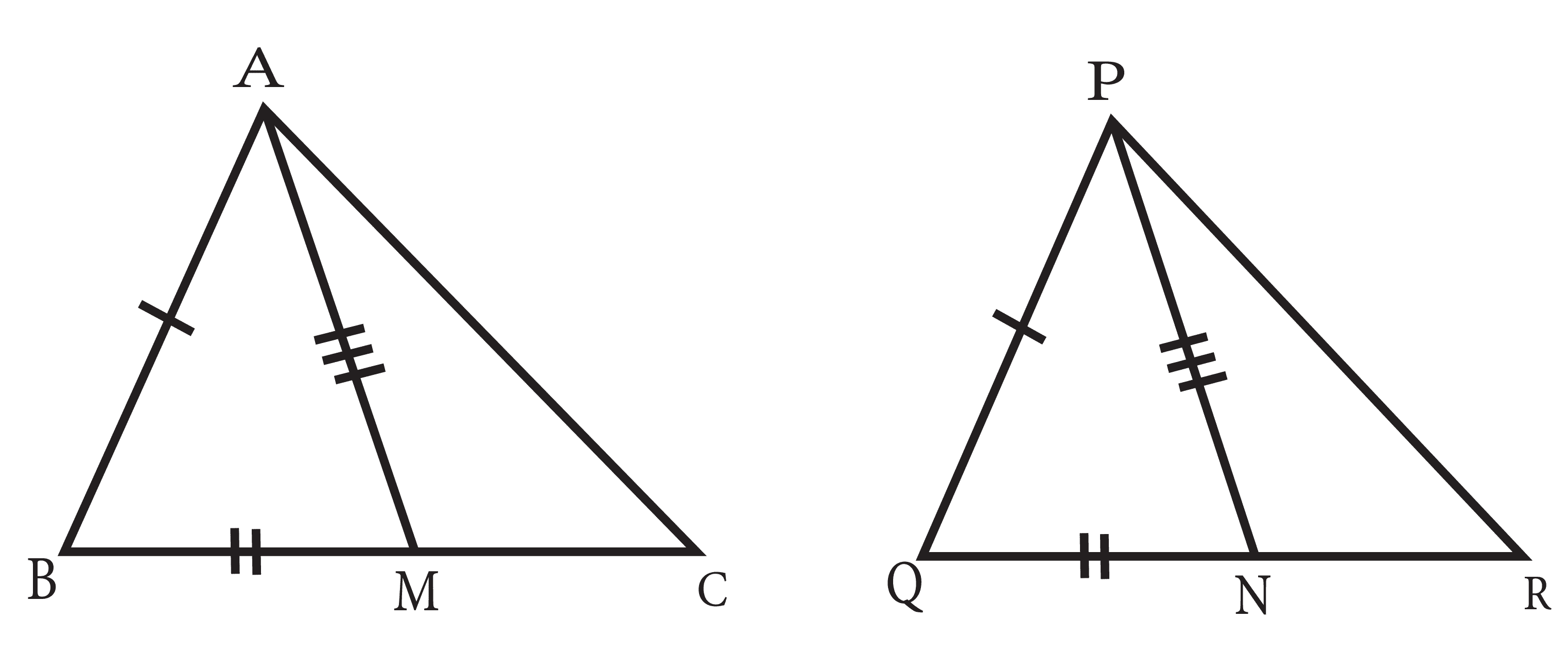(i) $\mathbf{\text{ }\!\!\Delta\!\!\text{ ABM}\cong \text{ }\!\!\Delta\!\!\text{ PQN}}$

Ans: In $\text{ }\!\!\Delta\!\!\text{ ABC}$, $\text{AM}$ is the median to $\text{BC}$.

Therefore, $\text{BM=}\frac{1}{2}BC$

In $\text{ }\!\!\Delta\!\!\text{ PQR}$, $PN$ is the median to $QR$.

Therefore, $\text{QN=}\frac{1}{2}QR$

However, $\text{BC=QR}$

So, $\text{BM=QN}$           …… (i)

In $\text{ }\!\!\Delta\!\!\text{ ABM}$ and $\text{ }\!\!\Delta\!\!\text{ PQN}$,

$\text{AB=PQ}$                                      (Given)

$\text{BM=QN}$                                    (From (i))

$\text{AM=PN}$                                     (Given)

Therefore, $\text{ }\!\!\Delta\!\!\text{ ABM}\cong \text{ }\!\!\Delta\!\!\text{ PQN}$       (By $SSS$ congruence rule)

$\angle ABM\text{=}\angle \text{PQN}$                         (By $\text{CPCT}$)

So, $\angle ABC\text{=}\angle \text{PQR}$              …… (ii)

(ii) $\mathbf{\text{ }\!\!\Delta\!\!\text{ ABC}\cong \text{ }\!\!\Delta\!\!\text{ PQR}}$

Ans: In $\text{ }\!\!\Delta\!\!\text{ ABC}$ and $\text{ }\!\!\Delta\!\!\text{ PQR}$.

$\text{AB=PQ}$                                      (Given)

$\angle ABC\text{=}\angle \text{PQR}$                        (from (ii))

$\text{BC=QR}$                                   (Given)

Therefore, $\text{ }\!\!\Delta\!\!\text{ ABC}\cong \text{ }\!\!\Delta\!\!\text{ PQR}$     (By $SAS$ congruence rule)

4. $\mathbf{BE}$ and $\mathbf{CF}$ are two equal altitudes of a triangle $\mathbf{ABC}$. Using $\mathbf{RHS}$ congruence rule, prove that the triangle $\mathbf{ABC}$ is isosceles.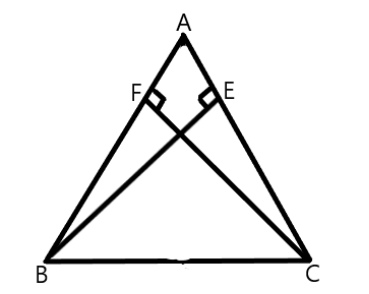Ans: In $\text{ }\!\!\Delta\!\!\text{ BEC}$ and $\text{ }\!\!\Delta\!\!\text{ CFB}$,

$\angle \text{BEC= }\angle \text{CFB}$                           (Each $\text{90 }\!\!{}^\text{o}\!\!\text{ }$)

$\text{BC=CB}$                                       (Common)

$\text{BE= CF}$                                       (Given)

Therefore, $\text{ }\!\!\Delta\!\!\text{ BEC}\cong \text{ }\!\!\Delta\!\!\text{ CFB}$          (By $RHS$ congruency)

Therefore, $\angle \text{BCE=}\angle \text{CBF}$           (By $\text{CPCT}$)

Therefore, $\text{AB=AC}$                     (Sides opposite to equal angles of a triangle are equal)

Hence, $\text{ }\!\!\Delta\!\!\text{ ABC}$ is isosceles.

5. $\mathbf{ABC}$ is an isosceles triangle with $\mathbf{\text{AB=AC}}$. Draw $\mathbf{\text{AP}\bot \text{BC}}$ to show that $\mathbf{\angle \text{B=}\angle \text{C}}$.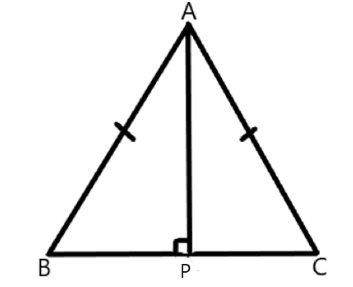Ans: In $\text{ }\!\!\Delta\!\!\text{ APB}$ and $\text{ }\!\!\Delta\!\!\text{ APC}$,

$\angle \text{APB = }\angle \text{APC}$                         (Each $\text{90 }\!\!{}^\text{o}\!\!\text{ }$)

$\text{AB=AC}$                                      (Given)

$\text{AP=AP}$                                       (Common)

Therefore, $\text{ }\!\!\Delta\!\!\text{ APB}\cong \text{ }\!\!\Delta\!\!\text{ APC}$         (Using $\text{RHS}$ congruence rule)

Therefore, $\angle \text{B=}\angle \text{C}$                    (By $\text{CPCT}$)

### Exercise (7.4)

1. Show that in a right angled triangle, the hypotenuse is the longest side.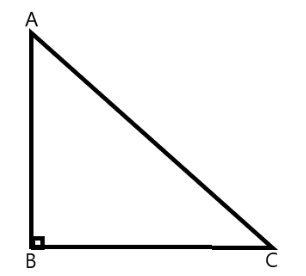Ans: Let us consider a right-angled triangle $\text{ABC}$, right-angled at $\text{B}$.

In $\text{ }\!\!\Delta\!\!\text{ ABC}$,

$\angle \text{A+}\angle \text{B +}\angle \text{C =180 }\!\!{}^\circ\!\!\text{ }$

$\angle \text{A+9}{{\text{0}}^{\text{o}}}\text{+}\angle \text{C =180 }\!\!{}^\circ\!\!\text{ }$

$\angle \text{A+}\angle \text{C =90 }\!\!{}^\circ\!\!\text{ }$

Hence, the other two angles have to be acute (i.e., less than $\text{90 }\!\!{}^\circ\!\!\text{ }$).

Let $\angle B\text{ =90 }\!\!{}^\circ\!\!\text{ }$

Since,

$\angle B>\angle A$

$\angle B>\angle C$

Therefore,

$AC<BC$

$AC<AB$                         (side opposite to the largest angle is longest)

Therefore, $AC$ is the largest side in $\text{ }\!\!\Delta\!\!\text{ ABC}$.

Hence, hypotenuse is the longest side in a right- angled triangle.

2. In the given figure sides $\mathbf{AB}$ and $\mathbf{AC}$ of $\mathbf{\text{ }\!\!\Delta\!\!\text{ ABC}}$ are extended to points $\mathbf{\text{P}}$ and $\mathbf{\text{Q}}$ respectively. Also, $\mathbf{\angle PBC<\angle QCB}$. Show that $\mathbf{AC>AB}$.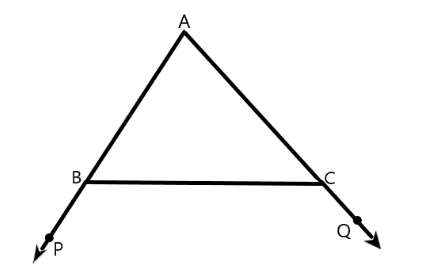Ans: In the given figure,

$\angle \text{ABC+}\angle \text{PBC=180 }\!\!{}^\circ\!\!\text{ }$                   (Linear pair)

$\angle \text{ABC= 180 }\!\!{}^\circ\!\!\text{ -}\angle \text{PBC}$                            …… (i)

Also,

$\angle \text{ACB+}\angle \text{QCB=180 }\!\!{}^\circ\!\!\text{ }$

$\angle \text{ACB= 180 }\!\!{}^\circ\!\!\text{ -}\angle \text{QCB}$                           …… (ii)

As

$\angle \text{PBC }\angle \text{QCB}$

$\text{180 }\!\!{}^\text{o}\!\!\text{ -}\angle \text{PBC}>\text{ 180 }\!\!{}^\text{o}\!\!\text{ - }\angle \text{QCB}$

$\angle \text{ABC }\angle \text{ACB}$                      (From Equations (i) and (ii))

Therefore, $\text{ACAB}$                (Side opposite to the larger angle is larger)

Hence proved, $\text{ACAB}$.

3. In the given figure, $\mathbf{\angle \text{B }\angle \text{A}}$ and $\mathbf{\angle \text{C}\angle \text{D}}$. Show that $\mathbf{\text{AD BC}}$.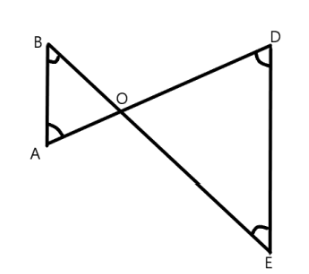Ans: In $\text{ }\!\!\Delta\!\!\text{ ABC}$

$\angle B\text{}\angle A$

Therefore, $\text{AOBO}$                      (Side opposite to smaller angle is smaller) ... (1)

In $\text{ }\!\!\Delta\!\!\text{ COD}$

$\angle \text{C}\angle D$

OD<OC                                      (Side opposite to smaller angle is smaller) ... (2)

On adding Equations (1) and (2), we obtain

$\text{AO +ODBO +OC}$

Hence proved.

4. $\mathbf{\text{AB}}$ and $\mathbf{\text{CD}}$ are respectively the smallest and longest sides of a quadrilateral $\mathbf{ABCD}$ (see the given figure). Show that $\angle A>\angle C$ and $\angle B>\angle D$.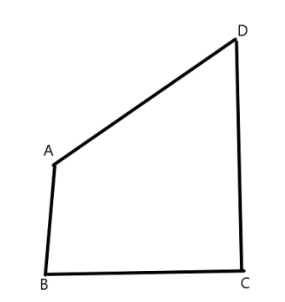Ans: Let us join $\text{AC}$.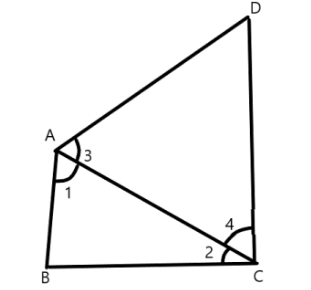In $\text{ }\!\!\Delta\!\!\text{ ABC}$,

$\text{AB < BC}$                       ($\text{AB}$ is smallest in quadrilateral $ABCD$)

$\angle \text{2}\angle 1$                        (Angle opposite to smaller side is smaller)…… (1)

In $\text{ }\!\!\Delta\!\!\text{ ABC}$,

$\text{AD < CD}$                     ($CD$ is largest in quadrilateral $ABCD$)

$\angle \text{4}\angle 3$                        (Angle opposite to smaller side is smaller)..... (2)

On adding Equations (1) and (2), we obtain

$\angle \text{2+}\angle \text{4}\angle \text{1+}\angle \text{3}$

$\angle \text{C}\angle \text{A}$

$\angle \text{A}\angle \text{C}$

Let us join $BD$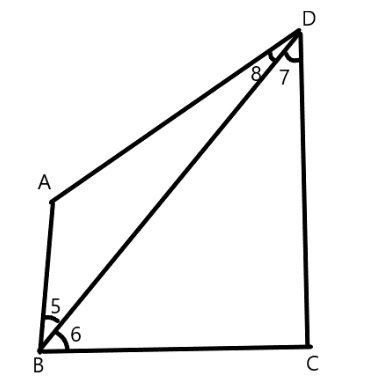In $\text{ }\!\!\Delta\!\!\text{ ABD}$,

$\text{AB < AD}$                       ($\text{AB}$ is smallest in quadrilateral $ABCD$)

$\angle 8\text{}\angle 5$                        (Angle opposite to smaller side is smaller)…… (3)

In $\text{ }\!\!\Delta\!\!\text{ BDC}$,

$\text{BC < CD}$                      ($CD$ is largest in quadrilateral $ABCD$)

$\angle 7\text{}\angle 6$                        (Angle opposite to smaller side is smaller)..... (4)

On adding Equations (3) and (4), we obtain

$\angle \text{8+}\angle \text{7}\angle \text{5+}\angle \text{6}$

$\angle \text{D }\angle \text{B}$

(Hence, proved)

5. In the given figure $\mathbf{\text{PR > PQ}}$ and $\mathbf{\text{PS}}$ bisects $\mathbf{\angle \text{QPR}}$. Prove that $\mathbf{\angle \text{PSR }\angle \text{PSQ}}$.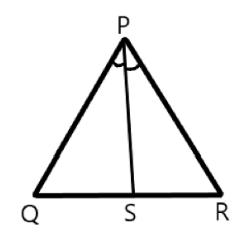Ans: As $\text{PR PQ}$,

$\angle \text{PQR}\angle \text{PRQ}$                           (Angle opposite to larger side is larger) ... (1)

$\text{PS}$ is the bisector of $\angle QPR$.

$\angle Q\text{PS=}\angle RPS$ ... (2)

$\angle PSR$ is the exterior angle of $\text{ }\!\!\Delta\!\!\text{ }PQS$.

$\angle PS\text{R=}\angle PQ\text{R +}\angle QPS$ ... (3)

$\angle PSQ$ is the exterior angle of $\text{ }\!\!\Delta\!\!\text{ }PRS$ .

$\angle \text{PSQ =}\angle \text{PRQ+ }\angle \text{RPS}$ ... (4)

Adding Equations (1) and (2), we obtain

$\angle \text{PQR+}\angle \text{QPS }\angle \text{PRQ+}\angle \text{RPS}$

$\angle \text{PSR}\angle \text{PSQ}$ (Using the values of Equations (3) and (4))

Hence proved.

6. Show that of all line segments drawn from a given point not on it, the perpendicular line segment is the shortest.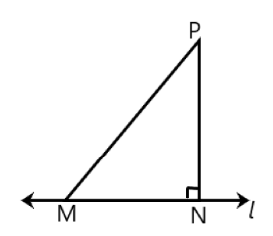Ans: Let us take a line $l$ and from point $P$ (i.e., not on line $l$), draw two line segments $PN$ and $PM$ . Let $PN$ be perpendicular to line $l$ and $PM$ is drawn at some other angle.

In $\text{ }\!\!\Delta\!\!\text{ }PNM$,

$\angle \text{N =90 }\!\!{}^\text{o}\!\!\text{ }$

$\angle \text{P +}\angle \text{N+ }\angle \text{M= 180 }\!\!{}^\text{o}\!\!\text{ }$                      (Angle sum property of a triangle)

$\angle \text{P+}\angle \text{M =90 }\!\!{}^\text{o}\!\!\text{ }$

Clearly, $\angle M$ is an acute angle.

$\angle \text{M}\angle N$

Therefore, $\text{PN < PM}$                          (Side opposite to the smaller angle is smaller)

Similarly, by drawing different line segments from $P$ to $l$ , it can be proved that $PN$ is smaller in comparison to them.

Therefore, it can be observed that of all line segments drawn from a given point not on it, the perpendicular line segment is the shortest.

### Exercise (7.5)

1. $\mathbf{\text{ABC}}$ is a triangle. Locate a point in the interior of $\mathbf{\text{ }\!\!\Delta\!\!\text{ ABC}}$ which is equidistant from all the vertices of

Ans: Circumcentre of a triangle is always equidistant from all the vertices of that triangle. Circumcentre is the point where perpendicular bisectors of all the sides of the triangle meet together.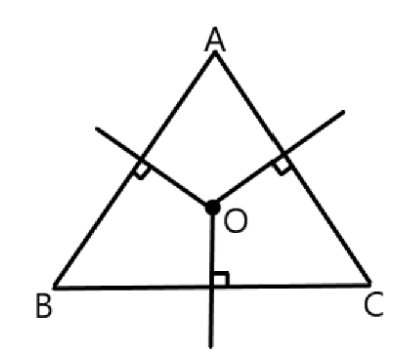In $\text{ }\!\!\Delta\!\!\text{ ABC}$, we can find the circumcentre by drawing the perpendicular bisectors of sides $\text{AB}$, $\text{BC}$, and $\text{CA}$ of this triangle. $\text{O}$ is the point where these bisectors are meeting together. Therefore, $\text{O}$ is the point which is equidistant from all the vertices of $\text{ }\!\!\Delta\!\!\text{ ABC}$

2. In a triangle locate a point in its interior which is equidistant from all the sides of the triangle.

Ans: The point which is equidistant from all the sides of a triangle is called the incentre of the triangle. Incentre of a triangle is the intersection point of the angle bisectors of the interior angles of that triangle.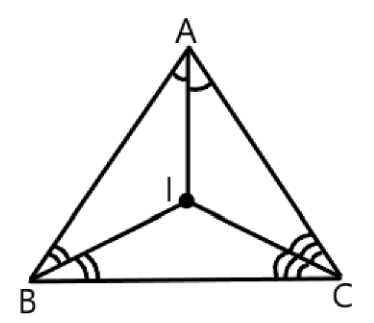Here, in $\text{ }\!\!\Delta\!\!\text{ ABC}$, we can find the incentre of this triangle by drawing the angle bisectors of the interior angles of this triangle. $\text{I}$ is the point where these angle bisectors are intersecting each other. Therefore, $\text{I}$ is the point equidistant from all the sides of $\text{ }\!\!\Delta\!\!\text{ ABC}$.

3. In a huge park people are concentrated at three points (see the given figure).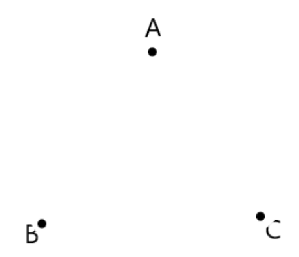$A$: Where there are different slides and swings for children,

$B$: Near which a man-made lake is situated,

$C$: Which is near to a large parking and exit.

Where should an ice-cream parlour be set up so that maximum number of persons can approach it?

(Hint: The parlor should be equidistant from $A$, $B$ and $C$)

Ans: Maximum number of persons can approach the ice-cream parlour if it is equidistant from $\text{A}$, $\text{B}$ and $\text{C}$. Now, $\text{A}$, $\text{B}$ and $\text{C}$ form a triangle. In a triangle, the circumcentre is the only point that is equidistant from its vertices. So, the ice-cream parlour should be set up at the circumcentre $\text{O}$ of

$\text{ }\!\!\Delta\!\!\text{ ABC}$.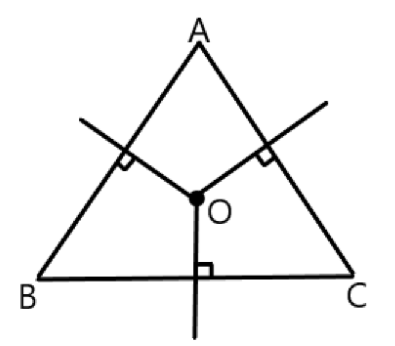In this situation, maximum number of persons can approach it. We can find circumcentre $\text{O}$ of this triangle by drawing perpendicular bisectors of the sides of this triangle.

4. Complete the hexagonal and star shaped rangolies (see the given figures) by filling them with as many equilateral triangles of side $\text{1cm}$ as you can. Count the number of triangles in each case. Which has more triangles?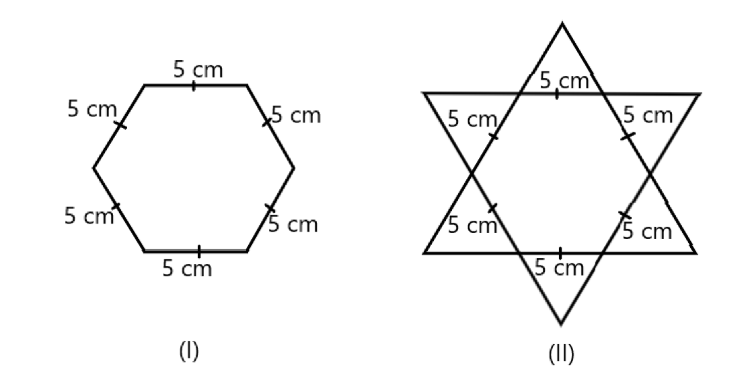Ans: It can be observed that hexagonal-shaped rangoli has $\text{6}$ equilateral triangles in it.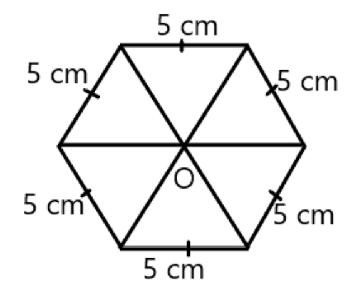Area of $\text{ }\!\!\Delta\!\!\text{ OAB=}\frac{\sqrt{\text{3}}}{\text{4}}{{\left( \text{Side} \right)}^{\text{2}}}$,

$\text{ }\!\!\Delta\!\!\text{ OAB=}\frac{\sqrt{\text{3}}}{\text{4}}{{\left( \text{5} \right)}^{\text{2}}}$

$\text{ }\!\!\Delta\!\!\text{ OAB=}\frac{\sqrt{\text{3}}}{\text{4}}\left( \text{25} \right)$

$\text{ }\!\!\Delta\!\!\text{ OAB=}\frac{\text{25}\sqrt{\text{3}}}{\text{4}}$

Area of hexagonal-shaped rangoli

$\text{=6 }\!\!\times\!\!\text{ }\frac{\text{25}\sqrt{\text{3}}}{\text{4}}$

$\text{=}\frac{\text{75}\sqrt{\text{3}}}{\text{2}}\text{c}{{\text{m}}^{\text{2}}}$

Area of equilateral triangle having its side as $\text{1cm}$

$\text{=}\frac{\sqrt{\text{3}}}{\text{4}}{{\left( \text{1} \right)}^{\text{2}}}$

$\text{=}\frac{\sqrt{\text{3}}}{\text{4}}\text{c}{{\text{m}}^{\text{2}}}$

Number of equilateral triangles of $\text{1cm}$ side that can be filled in this hexagonal-shaped rangoli

$\text{=}\frac{\frac{\text{75}\sqrt{\text{3}}}{\text{2}}}{\frac{\sqrt{\text{3}}}{\text{4}}}$

$\text{=150}$

Now, Star shaped rangoli has $\text{12}$ equilateral triangles of side $\text{5cm}$ in it: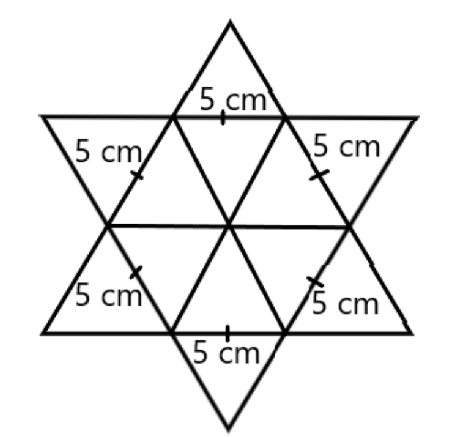Area of star-shaped rangoli

$\text{=12 }\!\!\times\!\!\text{ }\frac{\sqrt{\text{3}}}{\text{4}}\text{ }\!\!\times\!\!\text{ }{{\left( \text{5} \right)}^{\text{2}}}$

$\text{=75}\sqrt{\text{3}}$

Number of equilateral triangle of $\text{1cm}$ side that can be filled in this star-shaped rangoli

$\text{=}\frac{\frac{\text{75}\sqrt{\text{3}}}{\sqrt{\text{3}}}}{\text{4}}$

$\text{=300}$

Therefore, star-shaped rangoli has more equilateral triangle in it.

### NCERT Solutions For Class 9 Maths Chapter 7 Exercise 7.3- Free PDF Download

Create and maintain a robust foundation in all the topics of chapter 7 class Maths. NCERT Solutions for Class 9 maths chapter 7 exercise 7.3 provided by professionals are well-written with step-by-step explanations. These solutions come in handy so that students can rapidly finish their homework and class assessments. Since the interpretation of the questions is such a vital aspect when solving Maths problems, academicians pay special attention to make these solutions student-friendly.

### NCERT Solutions Class 9 Maths Chapter 7 Exercise 7.3

Class 9 maths exercise 7.3 educates students that a triangle is a closed figure formed by three lines intersecting. In the first section of the chapter, students will learn that if two figures possess the same shape and same size, then they are called congruent. Some of the examples include two squares of the same sides, two circles of the same radius, and two triangles whose corresponding parts are congruent. Theorems covered in class 9 maths ex 7.3 involve angles opposite to the equal sides and more.

Students will come across some congruence rules such as SAS, AAS, and ASA. SAS, which refers to Side- Angle- Side Congruence Rule states that if two sides and the comprised angle of one triangle are equal to the sides, and the formed angle of the other triangle, then two triangles are congruent. ASA, which refers to Angle- Side- Angle Congruence Rule, states that if two angles and the comprised side of one triangle are equal to two angles, and the formed side of the other triangle, then two triangles are congruent.

Apart from it, students will study the Side- Side- Side Congruence Rule in exercise 7.3 maths class 9. The rule states that two triangles are said to be congruent if three sides of one triangle are equal to the other triangle’s sides. Right angle- Hypotenuse- Side Congruence Rule states that two triangles are said to be congruent if the hypotenuse and one side of a triangle are equal to another triangle’s hypotenuse and its one side. Some of the inequalities in a triangle are also discussed in this section. Students should practice ample questions discussed in exercise 7.3 class 9 NCERT solutions for better understanding and marks.

### Class 9 Maths Chapter 7 Triangles Exercise 7.3- Weightage Marks

Triangles being a part of the Geometry unit hold a weightage of 22 marks out of 80 marks in class 9 Maths exam.

### Benefits of Class 9 Maths Chapter 7 Exercise 7.3 NCERT Solutions

NCERT solutions help students to provide a strong foundation over the concepts covered in exercise 7.3. Answering the NCERT questions, require in-depth knowledge along with a better understanding of formulae. Thus, it’s preferable to check expert-written solutions following the correct approach for every question given in the exercise. Some of the benefits of considering NCERT solutions for class 9 maths chapter 7 exercise 7.3 include:

• Every question and answer discussed are provided by a professional expert with an all-inclusive explanation.

• Maths NCERT solutions class 9 chapter 7 exercise 7.3 solutions help students to select a higher-grade stream according to their interest.

• NCERT solutions offered by Vedantu are well-prepared according to the guidelines of the CBSE board.

• By practicing NCERT exercise 7.3 solutions, students can find it hassle-free to solve questions asked from the chapter Triangles in exams effortlessly.

• The students can score a good percentage in their annual examinations after referring through these solutions.

## FAQs on NCERT Solutions for Class 9 Maths Chapter 7 - Exercise

1. How to show AB bisects PQ if AB is an altitude of an isosceles triangle APQ in which AP = AQ?

In the question, it’s given that AB is an altitude and AP = AQ. Now, in a triangle, APB and AQB, angle ABP = 90o and ABQ = 90o. As AP = AQ and AB is the common arm in the triangle APB and AQB, then apply the RHS congruence rule. The rule states that two triangles are said to be congruent if the hypotenuse and one side of a triangle are equal to another triangle’s hypotenuse and its one side. Hence, both the triangles APB and AQB are equal. Now, by applying the CPCT rule, PB is equal to QB. Hence, AB bisects PQ.

2. How to prove that the ABC is an isosceles triangle if BE and CF are two altitudes equal to each other?

Given that BE and CF are two altitudes of an isosceles triangle that are equal to each other. In the triangle BEC and CFB, angle BEC is equal to 90o and CFB is equal to 90o. Also, BC = CB as it is a common side. Similarly, BE and CF are equal. By applying the RHS Congruence Rule, triangle BEC and CFB are equal to each other. Additionally, by CPCT rule, C = B. It means that sides opposite to the equal angles are equal always. Hence, AB = AC. It shows, that side AB and BC of the triangle ABC form an isosceles triangle.

3. What is a Triangle, and what are its properties?

A triangle is a type of polygon with three sides. It is a closed, two-dimensional shape made of straight lines. Therefore every triangle has a total of three vertices, and each vertex forms an angle. The main properties of a triangle are as follows.

• It has three sides and therefore three angles, and the sum of the three angles is 180 degrees.

• The addition of all the exterior angles of a triangle is 360 degrees.

• The total sum of the lengths of any two sides of a triangle is invariably greater than the length of the third side.

• The shortest side of the triangle always lies opposite to the smallest interior angle.

4. How many sums are there in Exercise 7.3 of Class 9 Maths?

There are a total of five sums in Class 9 Maths Chapter 7 Exercise 7.3. Each sum assesses the application of the concepts of triangles and their various properties. The sums are based on the understanding of the properties of a triangle, the congruence of a triangle, rules of congruences, the theorems and corollaries of inequalities of triangles, and many more. In the NCERT Solutions of Class 9 Maths Chapter 7 Ex-7.3 PDF, every sum is followed by a detailed analysis of self-explanatory solutions helping students get a better understanding of the concepts.

5. Is Exercise 7.3 of 9th Maths easy?

Yes, if practised and understood well, this exercise is extremely easy for the students. In order to get help in comprehending the concepts, the students should get help from the NCERT solutions. These solutions have all the exercises with detailed and explanatory answers that will help the student in understanding the concepts in a better light. With the practice of this exercise from the NCERT solutions, the student will attain confidence in their knowledge, which will eventually help them in scoring better marks.

6. What is the importance of this exercise in Mathematics?

Triangles are one of the first shapes that are studied under geometry. They are important in academics because arbitrary polygons with 4, 5, 6, or n sides can be usually decomposed into triangles. Therefore, the basic properties of a triangle will pave the way for learning and analysing a much deeper study of the other polygons. This exercise caters to this basic knowledge of a triangle, and some major concepts such as the Pythagoras Theorem and trigonometry are dependent on the properties of a triangle.

7. Is this the only exercise that deals with the concept of Triangles?

There are a total of 5 sections that deal with different concepts within the study of Triangles. Each of these sections has varied problems based on different theories and formulas, and without the understanding of the previous section, it becomes difficult to get a better hold of the successive exercise. Mastery of each of these sections will help score better marks, as the questions usually asked in the examination are not always straightforward. They are rather a blend of all these concepts present in the various exercises.